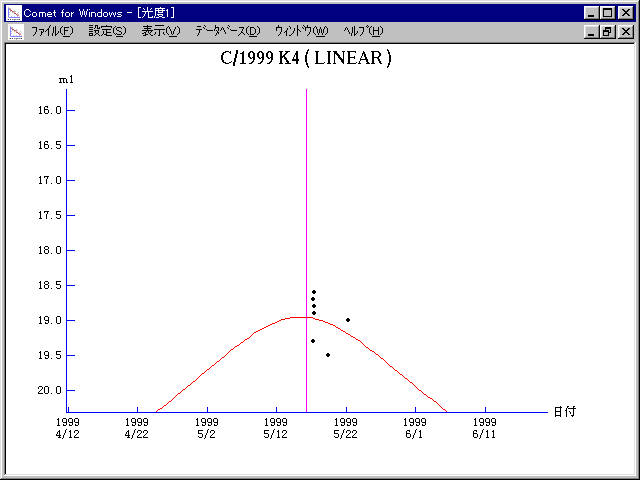# \$B%j%K%"WB@1(B

C/1999 K4 ( LINEAR )###\$B%W%m%U%#!<%k(B

 \$BH/8+F|(B 1999\$BG/(B 5\$B7n(B17\$BF|(B \$BH/8+8wEY(B 18.7\$BEy(B \$BH/8+ Lincoln Laboratory Near-Earth Asteroid Research project

###\$B50F;MWAG(B

```    Orbital elements by K. Muraoka,
from 17 observations   1999 May 17 to 22,
m.e. =  +/- 0.71 arc seconds.

T  =  1999 May  16.337  TT
Peri. =    8.832
Node  =  241.637   (2000.0)
Incl. =  121.310
q  =    1.44831  AU
e  =    1.0
```

###\$B@1?^(B###\$B8wEYJQ2=(B

```        m1 = 19.0 + 5 log\$B&\$(B + 10.0 log r
```##### \$B50F;MWAG\$OB<2,7r<#;a\$N7W;;\$K\$h\$k\$b\$N\$G\$9!#(B \$B@1?^\$O(B StellaNavigator Ver.2.0 for Windows (\$B%"%9%H%m%"!<%D(B \$BJTCx(B / \$B%"%9%-!<=PHG6I4)(B) \$B\$G:n@.\$7\$?\$b\$N\$G\$9!#(B \$B8wEY%0%i%U\$O(BComet for Windows\$B\$G:n@.\$7\$?\$b\$N\$G\$9!#(B